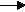gcsescience.com                                       26                                       gcsescience.com

Moles

Masses or Volumes from Electrolysis.

A unit of electrical charge is called a coulomb.
One coulomb (symbol Q) is 6·2 x 1018 electrons.

One mole of electrons is the same as 96,500 coulombs.
96,500 coulombs is called a faraday.

Electric current (symbol I) is a flow of electrical charge.
The rate of flow of electrical charge is measured in amps (A).
One amp is one coulomb per second.    Q = I x t.

Example 3
.

The electrolysis of molten lead bromide - PbBr2
produces lead at the cathode and bromine at the anode.

A current of 10 amps is allowed to flow
through molten lead bromide for 5 hours.
What mass of lead is deposited at the cathode?

Method.
1) Write the half equation for the electrolysis.
Pb2+  +    2e-Pb
2 moles of electrons (2 faradays)
are required to deposit 1 mole of lead.

2) Find how many faradays have passed through
the lead bromide in 5 hours.

Q = I x t

5 hours contains (5 x 60 x 60) seconds
= 18,000 seconds.

Q = 10 x 18,000
= 180,000 coulombs.

180,000 coulombs = 180,000 ÷ 96,500 faradays

3) From the proportion in 1 above,

mass = moles x RAM